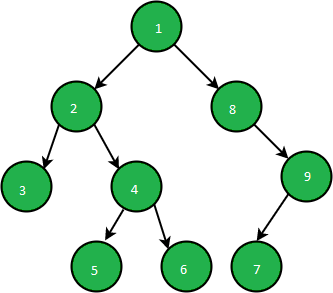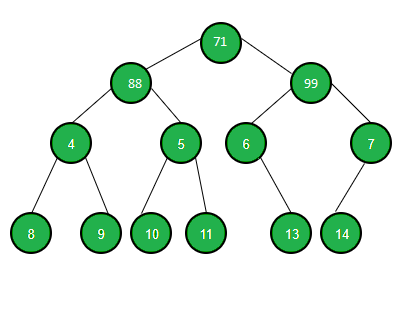# Print alternate nodes from all levels of a Binary Tree

Given a binary tree, the task is to traverse each level of the given binary tree from left to right and print every alternate encountered at a level.

Examples:

Input:Output:

3 9
5 7

Input:Output:
71
88
4 6
8 10 13

Approach: The problem can be solved by performing Level Order Traversal traversal on the given binary tree. Follow the steps below to solve the problem:

1. Initialize a Queue, to store the nodes of each level of the given binary tree.
2. Perform level order traversal and print alternate nodes from each level of the Binary Tree

Below is the implementation of the above approach:

## C++

 `// C++ program to implement` `// the above approach`   `#include ` `using` `namespace` `std;`   `// Structure of a Node` `struct` `Node {` `    ``int` `data;` `    ``Node* left;` `    ``Node* right;` `    ``Node(``int` `val)` `    ``{` `        ``data = val;` `        ``left = right = NULL;` `    ``}` `};`   `// Print alternate nodes of` `// a binary tree` `void` `PrintAlternate(Node* root)` `{` `    ``// Store nodes of each level` `    ``queue Q;` `    ``Q.push(root);`   `    ``while` `(!Q.empty()) {` `        ``// Store count of nodes` `        ``// of current level` `        ``int` `N = Q.size();`   `        ``// Print alternate nodes of` `        ``// the current level` `        ``for` `(``int` `i = 0; i < N; i++) {` `            ``Node* temp = Q.front();` `            ``Q.pop();`   `            ``if` `(i % 2 == 0) {` `                ``cout << temp->data << ``" "``;` `            ``}`   `            ``// If left child exists` `            ``if` `(temp->left) {` `                ``// Store left child` `                ``Q.push(temp->left);` `            ``}`   `            ``// If right child exists` `            ``if` `(temp->right) {` `                ``// Store right child` `                ``Q.push(temp->right);` `            ``}` `        ``}` `        ``cout << endl;` `    ``}` `}`   `// Driver Code` `int` `main()` `{` `    ``Node* root;`   `    ``// Create a tree` `    ``root = ``new` `Node(71);` `    ``root->left = ``new` `Node(88);` `    ``root->right = ``new` `Node(99);` `    ``root->left->left = ``new` `Node(4);` `    ``root->left->right = ``new` `Node(5);` `    ``root->right->left = ``new` `Node(6);` `    ``root->right->right = ``new` `Node(7);` `    ``root->left->left->left = ``new` `Node(8);` `    ``root->left->left->right = ``new` `Node(9);` `    ``root->left->right->left = ``new` `Node(10);` `    ``root->left->right->right = ``new` `Node(11);` `    ``root->right->left->right = ``new` `Node(13);` `    ``root->right->right->left = ``new` `Node(14);` `    ``PrintAlternate(root);` `}`

## Java

 `// Java program to implement` `// the above approach` `import` `java.util.*;` `class` `GFG{`   `// Structure of a Node` `static` `class` `Node ` `{` `  ``int` `data;` `  ``Node left;` `  ``Node right;` `  ``Node(``int` `val)` `  ``{` `    ``data = val;` `    ``left = right = ``null``;` `  ``}` `};`   `// Print alternate nodes of` `// a binary tree` `static` `void` `PrintAlternate(Node root)` `{` `  ``// Store nodes of each level` `  ``Queue Q = ``new` `LinkedList<>();` `  ``Q.add(root);`   `  ``while` `(!Q.isEmpty()) ` `  ``{` `    ``// Store count of nodes` `    ``// of current level` `    ``int` `N = Q.size();`   `    ``// Print alternate nodes of` `    ``// the current level` `    ``for` `(``int` `i = ``0``; i < N; i++) ` `    ``{` `      ``Node temp = Q.peek();` `      ``Q.remove();`   `      ``if` `(i % ``2` `== ``0``) ` `      ``{` `        ``System.out.print(temp.data + ``" "``);` `      ``}`   `      ``// If left child exists` `      ``if` `(temp.left!=``null``) ` `      ``{` `        ``// Store left child` `        ``Q.add(temp.left);` `      ``}`   `      ``// If right child exists` `      ``if` `(temp.right!=``null``) ` `      ``{` `        ``// Store right child` `        ``Q.add(temp.right);` `      ``}` `    ``}` `    ``System.out.println();` `  ``}` `}`   `// Driver Code` `public` `static` `void` `main(String[] args)` `{` `  ``Node root;`   `  ``// Create a tree` `  ``root = ``new` `Node(``71``);` `  ``root.left = ``new` `Node(``88``);` `  ``root.right = ``new` `Node(``99``);` `  ``root.left.left = ``new` `Node(``4``);` `  ``root.left.right = ``new` `Node(``5``);` `  ``root.right.left = ``new` `Node(``6``);` `  ``root.right.right = ``new` `Node(``7``);` `  ``root.left.left.left = ``new` `Node(``8``);` `  ``root.left.left.right = ``new` `Node(``9``);` `  ``root.left.right.left = ``new` `Node(``10``);` `  ``root.left.right.right = ``new` `Node(``11``);` `  ``root.right.left.right = ``new` `Node(``13``);` `  ``root.right.right.left = ``new` `Node(``14``);` `  ``PrintAlternate(root);` `}` `}`   `// This code is contributed by 29AjayKumar`

## C#

 `// C# program to implement` `// the above approach` `using` `System;` `using` `System.Collections.Generic;` `class` `GFG{`   `// Structure of a Node` `class` `Node ` `{` `  ``public` `int` `data;` `  ``public` `Node left;` `  ``public` `Node right;` `  ``public` `Node(``int` `val)` `  ``{` `    ``data = val;` `    ``left = right = ``null``;` `  ``}` `};`   `// Print alternate nodes of` `// a binary tree` `static` `void` `PrintAlternate(Node root)` `{` `  ``// Store nodes of each level` `  ``Queue Q = ``new` `Queue();` `  ``Q.Enqueue(root);`   `  ``while` `(Q.Count != 0) ` `  ``{` `    ``// Store count of nodes` `    ``// of current level` `    ``int` `N = Q.Count;`   `    ``// Print alternate nodes of` `    ``// the current level` `    ``for` `(``int` `i = 0; i < N; i++) ` `    ``{` `      ``Node temp = Q.Peek();` `      ``Q.Dequeue();`   `      ``if` `(i % 2 == 0) ` `      ``{` `        ``Console.Write(temp.data + ``" "``);` `      ``}`   `      ``// If left child exists` `      ``if` `(temp.left!=``null``) ` `      ``{` `        ``// Store left child` `        ``Q.Enqueue(temp.left);` `      ``}`   `      ``// If right child exists` `      ``if` `(temp.right!=``null``) ` `      ``{` `        ``// Store right child` `        ``Q.Enqueue(temp.right);` `      ``}` `    ``}` `    ``Console.WriteLine();` `  ``}` `}`   `// Driver Code` `public` `static` `void` `Main(String[] args)` `{` `  ``Node root;`   `  ``// Create a tree` `  ``root = ``new` `Node(71);` `  ``root.left = ``new` `Node(88);` `  ``root.right = ``new` `Node(99);` `  ``root.left.left = ``new` `Node(4);` `  ``root.left.right = ``new` `Node(5);` `  ``root.right.left = ``new` `Node(6);` `  ``root.right.right = ``new` `Node(7);` `  ``root.left.left.left = ``new` `Node(8);` `  ``root.left.left.right = ``new` `Node(9);` `  ``root.left.right.left = ``new` `Node(10);` `  ``root.left.right.right = ``new` `Node(11);` `  ``root.right.left.right = ``new` `Node(13);` `  ``root.right.right.left = ``new` `Node(14);` `  ``PrintAlternate(root);` `}` `}`   `// This code is contributed by 29AjayKumar`

Output:

```71
88
4 6
8 10 13

```

Time Complexity: O(N)
Auxiliary Space: O(N)

Attention reader! Don’t stop learning now. Get hold of all the important DSA concepts with the DSA Self Paced Course at a student-friendly price and become industry ready.

My Personal Notes arrow_drop_upCheck out this Author's contributed articles.

If you like GeeksforGeeks and would like to contribute, you can also write an article using contribute.geeksforgeeks.org or mail your article to contribute@geeksforgeeks.org. See your article appearing on the GeeksforGeeks main page and help other Geeks.

Please Improve this article if you find anything incorrect by clicking on the "Improve Article" button below.

Improved By : 29AjayKumar